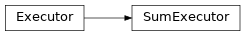# SumExecutor¶

Inheritance Diagramclass ashpy.losses.executor.SumExecutor(executors)[source]

The sum executor. Executes the call of each fn and weights the losses.

Each Executor gets called (thus reducing its carried function), the results are then summed together.

Methods

 __init__(executors) Initialize the SumExecutor. call(*args, **kwargs) Evaluate and sum together the Executors.

Attributes

 executors Return the List of Executors. fn Return the Keras loss function to execute. global_batch_size Global batch size comprises the batch size for each cpu. weight Return the loss weight.
__init__(executors)[source]

Initialize the SumExecutor.

Parameters: executors (list of [ashpy.executors.Executor]) – Array of ashpy.executors.Executor to sum evaluate and sum together. None None
call(*args, **kwargs)[source]

Evaluate and sum together the Executors.

Return type: Tensor :py:classes:tf.Tensor – Output Tensor.
executors

Return the List of Executors.

Return type: List[Executor]
global_batch_size

Global batch size comprises the batch size for each cpu.

Calculated as batch_size_for_replica*replica_numbers.

Return type: int int – Global Batch size value.